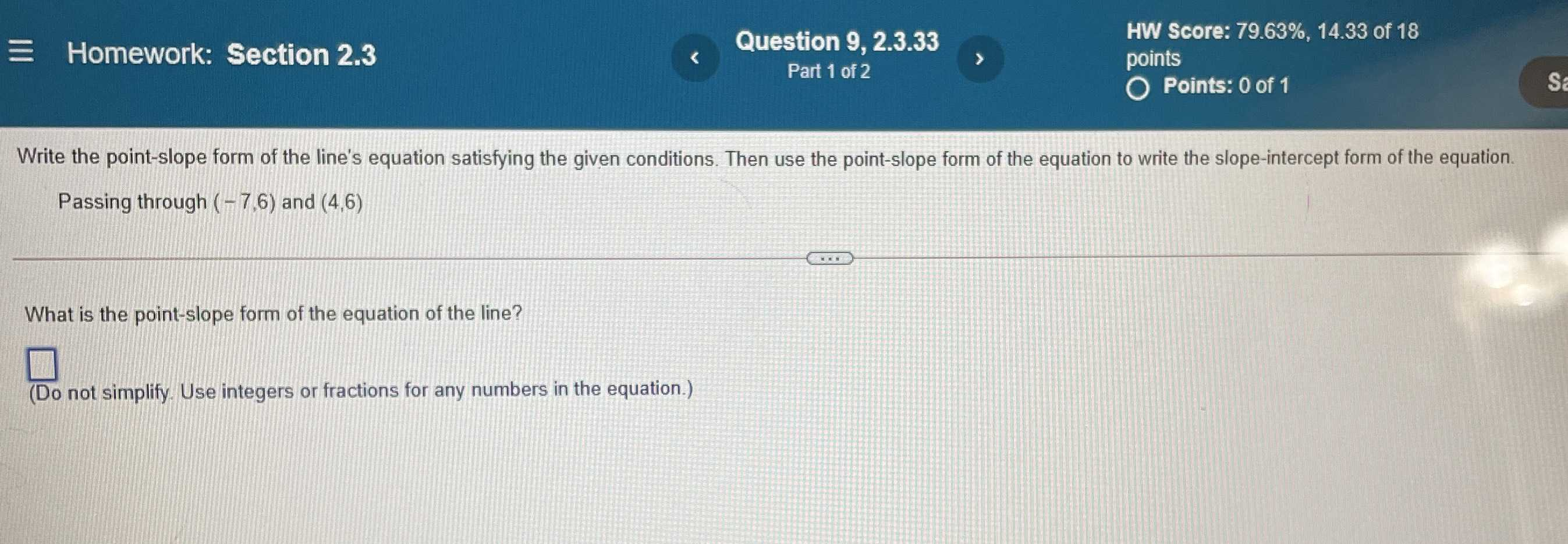### ¿Todavía tienes preguntas de matemáticas?

Pregunte a nuestros tutores expertos
Algebra
PreguntaWrite the point-slope form of the line's equation satisfying the given conditions. Then use the point-slope form of the equation to write the slope-intercept form of the equation. Passing through $$( - 7,6 )$$ and $$( 4,6 )$$ What is the point-slope form of the equation of the line?

(Do not simplify. Use integers or fractions for any numbers in the equation.)